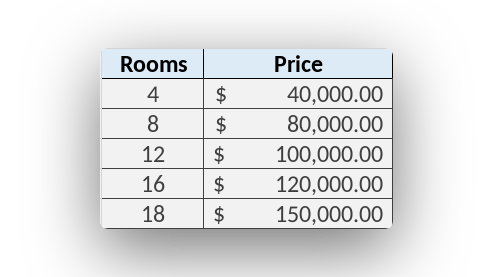Linear regression algorithm predicts continous values (like price, temperature).
This is another article in the machine learning algorithms for beginners series.
It is a supervised learning algorithm, you need to collect training data for it to work.

Related course: Python Machine Learning Course

## Linear Regression

### Introduction

Classification output can only be discrete values. There can be ,, etcetera.
What if you want to output prices or other continous values?

Then you use a regression algorithm.

Lets say you want to predict the housing price based on features. Collecting data is the
first step. Features could be number of rooms, area in m^2, neighborhood quality and others.### Example

Write down the feature: #area_m2.
For our example in code that looks like this.

Then you can create a plot based on that data (if you want to).
You see there is a correlation between the area and the price.

This is a linear relationship.
You can predict the price, with a linear regression algorithm.

If you are new to Machine Learning, then I highly recommend this book.

### Explanation

First you import the linear regression algorithm from like it learn then you defined a training data X and the Y where axis the area and y is the price.

Linear regression algorithm because there is a linear relationship then we train the algorithm using the training data.

Now that the algorithm is trained you can make predictions using the area.
A new example, can predict the price for you.

This algorithm LinearRegression only works if there is a linear relation in your data set.
If there isn’t, you need a polynomial algorithm.

Plot to verify that there is a linear relation.